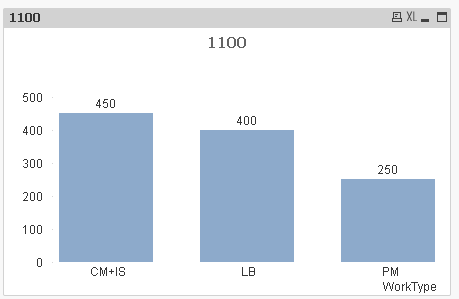# QlikView Scripting

Discussion Board for collaboration on QlikView Scripting.

HighlightedNot applicable

## How to combine rows or columns of excel data in qlik

Let's say I have a spreadsheet that looks something like this:

WO#Work TypeLABCOSTOHCOST

111

PM10050
112PM0100
113CM200200
114LB0400
115IS500

If I wanted to make a bar graph that compares the sum of the costs of each Work Type, how can I make qlikview sum LABCOST and OHCOST without making another column in my spreadsheet (so the sum of the costs would be 250 for PM, 400 for CM, etc.). Also, How could I combine CM and IS in qlikview so when I made a similar bar graph it would show the costs for PM, LB, and a third Work Type that is a combo of CM and IS? My spreadsheet has >500,000 rows of data, so making these changes in excel would be a pain...

Tags (2)
1 Solution

Accepted Solutions
Esteemed Contributor III

## Re: How to combine rows or columns of excel data in qlik

Something like this?

Load the data from Excel File like below change the path accordingly.

WO#, WorkType, LABCOST, OHCOST

FROM Excelfilename.xlsx;

Create a bar chart :

Dim: = IF(Match(WorkType, 'CM', 'IS'), 'CM+IS', WorkType)

Expr: = = Sum( LABCOST + OHCOST)4 Replies
Esteemed Contributor III

## Re: How to combine rows or columns of excel data in qlik

Something like this?

Load the data from Excel File like below change the path accordingly.

WO#, WorkType, LABCOST, OHCOST

FROM Excelfilename.xlsx;

Create a bar chart :

Dim: = IF(Match(WorkType, 'CM', 'IS'), 'CM+IS', WorkType)

Expr: = = Sum( LABCOST + OHCOST)MVP & Luminary

## Re: How to combine rows or columns of excel data in qlik

 If I wanted to make a bar graph that compares the sum of the costs of each Work Type, how can I make qlikview sum LABCOST and OHCOST

Sum(RangeSum(LABCOST,OHCOST))

 How could I combine CM and IS in qlikview

Create a new table with a new dimension:

WorkTypeGroup, Work Type

PM, PM

CM, CM

CM, IS

LB, LB

];

Then use the new WorkTypeGroup as dimension instead of [Work Type]

talk is cheap, supply exceeds demandNot applicable

## Re: How to combine rows or columns of excel data in qlik

New question that is kinda related to the last one. I have a very large excel sheet that I am loading into qlik. There is a column heading called "PERSONGROUP" that has about 50-60 different inputs which should really be grouped into about 10-15 input groups (i.e. 'IC', 'IC1', 'IC2', 'IC3' should be 'IC' and 'FAC', 'FAC1' should be 'FAC', etc.). What do I need to do so that all of these inputs are grouped together the way I want them to be? I tried using multiple dimensions with the =if(match(...)) that Vish suggested to use in the chart script, but that only worked for combining one group...

Esteemed Contributor III

## Re: How to combine rows or columns of excel data in qlik

Ok Use what gysbert suggested like create an Inline table with all mapping then use that the dimensions as

WorkTypeGroup removing If(Match...()).